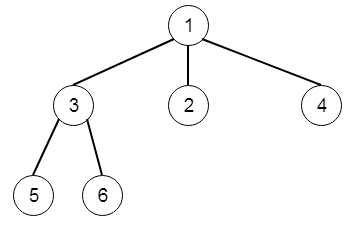# N-ary Tree Level Order Traversal in C++

Suppose we have an n-ary tree, we have to return the level order traversal of its nodes' values. The Nary-Tree input serialization is represented in their level order traversal. Here each group of children is separated by the null value (See examples). So the following tree can be represented as [1,null,3,2,4,null,5,6]The output will be [,[3,2,4],[5,6]]

To solve this, we will follow these steps −

• make one matrix ans

• if root is null, return ans

• make one queue q and insert root

• while q is not empty

• size := size of the queue

• make one array temp

• while size is not 0

• curr := first element of q

• insert value of curr into temp

• delete element from queue

• for i in range 0 to size of the children of curr

• insert ith children of curr

• decrease size by 1

• insert temp into ans

• return ans

Let us see the following implementation to get better understanding −

## Example

Live Demo

#include <bits/stdc++.h>
using namespace std;
void print_vector(vector<vector<auto> > v){
cout << "[";
for(int i = 0; i<v.size(); i++){
cout << "[";
for(int j = 0; j <v[i].size(); j++){
cout << v[i][j] << ", ";
}
cout << "],";
}
cout << "]"<<endl;
}
class Node {
public:
int val;
vector<Node*> children;
Node() {}
Node(int _val) {
val = _val;
}
Node(int _val, vector<Node*> _children) {
val = _val;
children = _children;
}
};
class Solution {
public:
vector<vector<int>> levelOrder(Node* root) {
vector < vector <int> > ans;
if(!root)return ans;
queue <Node*> q;
q.push(root);
while(!q.empty()){
int sz = q.size();
vector<int> temp;
while(sz--){
Node* curr = q.front();
temp.push_back(curr->val);
q.pop();
for(int i = 0; i < curr->children.size(); i++){
q.push(curr->children[i]);
}
}
ans.push_back(temp);
}
return ans;
}
};
main(){
Node *root = new Node(1);
Node *left_ch = new Node(3), *mid_ch = new Node(2), *right_ch = new Node(4);
left_ch->children.push_back(new Node(5));
left_ch->children.push_back(new Node(6));
root->children.push_back(left_ch);
root->children.push_back(mid_ch);
root->children.push_back(right_ch);
Solution ob;
print_vector(ob.levelOrder(root));
}

## Input

[1,null,3,2,4,null,5,6]

## Output

[[1, ],[3, 2, 4, ],[5, 6, ],]